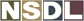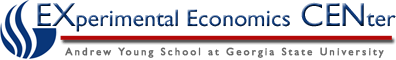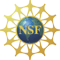Students / Subjects# Choices and Preferences

A set of alternatives, such as those in figure 1, restricts a consumer's set of possible choices. If a consumer has stable preferences, then consistent patterns of choice will emerge when the set of alternatives changes. An important example of a systematic change to the set of alternative choices occurs when the price of a commodity changes. One important objective of models of preferences and demand is to evaluate patterns of choice as prices change.

The previous section described the consumer's budget, which creates a set of alternatives from which a choice is made. This section examines a hypothetical choice example. After the choice example, the idea of preferences is introduced.

Preference is a very natural and intuitive notion. A consumer, presented with two alternatives, should be able to decide which is preferred, or if they are equally good. The idea of a preference relation formalizes this idea, and extends it so that preferences are defined for all alternatives. Once the idea of preferences is developed, we can ask what properties we expect preferences to have, and finally, conditions on preferences that insure that preferences can be represented by a utility function.

Preferences are natural psychologically, but most analysis of consumer behavior is based on utility functions. A utility function assigns a "value" to each alternative choice, and the predicted choice is the alternative with the highest of these values. Utility functions are useful for analysis, since demand functions can be derived from them.

This section describes these topics in succession, following the outline below.